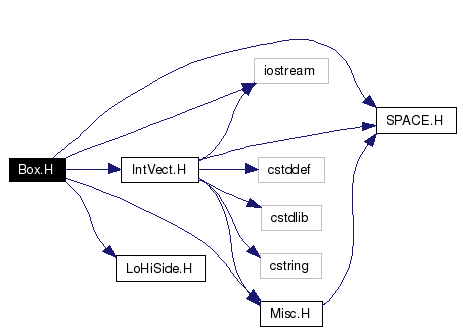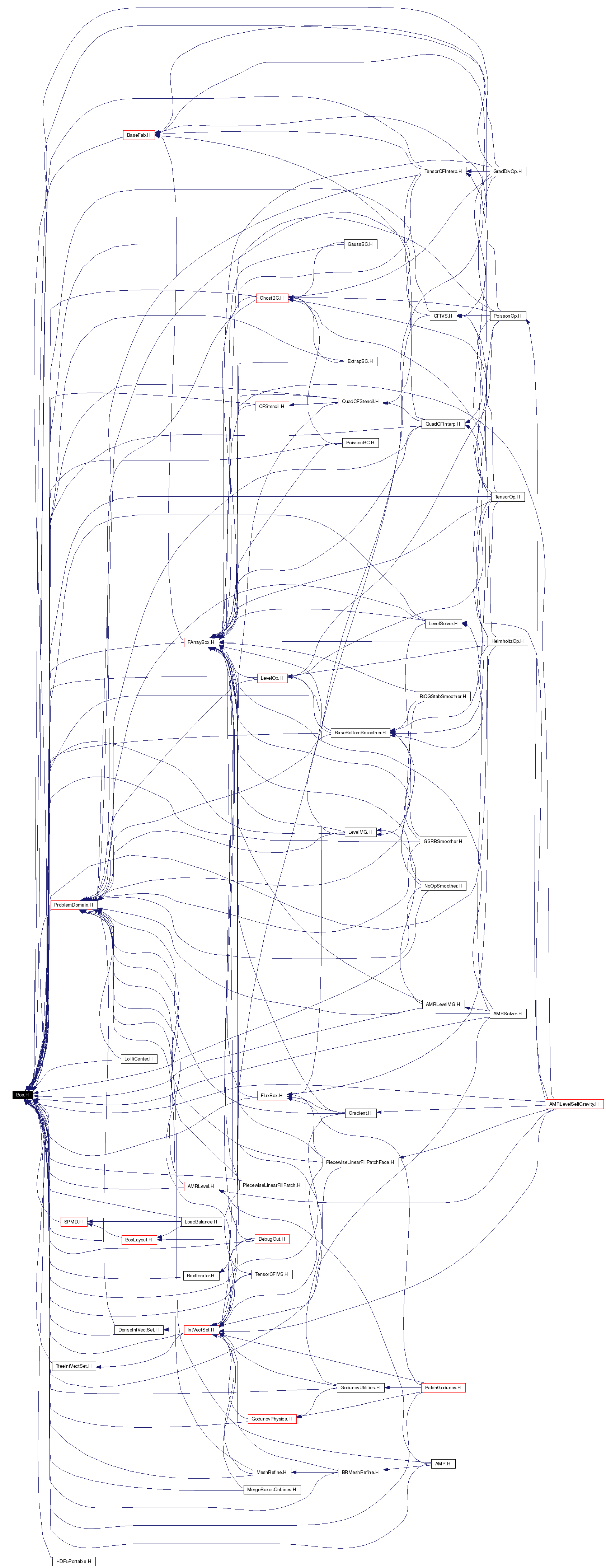# Box.H File Reference

`#include "SPACE.H"`
`#include <iostream>`
`#include "IntVect.H"`
`#include "Misc.H"`
`#include "LoHiSide.H"`

Include dependency graph for Box.H:This graph shows which files directly or indirectly include this file:Go to the source code of this file.

## Functions

Box surroundingNodes (const Box &b, int dir)
Box surroundingNodes (const Box &b)
Box enclosedCells (const Box &b, int dir)
Box enclosedCells (const Box &b)
Box bdryBox (const Box &b, int dir, Side::LoHiSide a_sd, int len=1)
Box bdryLo (const Box &b, int dir, int len=1)
Box bdryHi (const Box &b, int dir, int len=1)
Box adjCellLo (const Box &b, int dir, int len=1)
Box adjCellHi (const Box &b, int dir, int len=1)
Box minBox (const Box &b1, const Box &b2)
Box coarsen (const Box &b, const IntVect &refinement_ratio)
Box coarsen (const Box &b, int refinement_ratio)
Box refine (const Box &b, const IntVect &refinement_ratio)
Box refine (const Box &b, int refinement_ratio)
Box grow (const Box &b, int i)
Box grow (const Box &b, const IntVect &v)

## Function Documentation

 Box adjCellHi ( const Box & b, int dir, int len = `1` )
 Returns the cell centered Box of the given length adjacent to the argument Box on the high end along the given coordinate direction. The return Box is identical to the argument Box in the other directions. The return Box and the argument Box have an empty intersection. \ NOTE: len >= 1. \ NOTE: Box retval = adjCellHi(b,dir,len) is equivalent to the following set of operations: \ Box retval(b); \ retval.convert(dir,Box::CELL); \ retval.setrange(dir,retval.bigEnd(dir)+1,len);\ Directions are zero-based. It is an error if not 0 <= dir < SpaceDim. The neighbor of an Empty Box is an Empty Box of the appropriate type.

 Box adjCellLo ( const Box & b, int dir, int len = `1` )
 Returns the cell centered Box of the given length adjacent to the argument Box on the low end along the given coordinate direction. The return Box is identical to the argument Box in the other directions. The return Box and the argument Box have an empty intersection. \ NOTE: len >= 1. \ NOTE: Box retval = adjCellLo(b,dir,len) is equivalent to the following set of operations: \ Box retval(b); \ retval.convert(dir,Box::CELL); \ retval.setrange(dir,retval.smallEnd(dir)-len,len); \ Directions are zero-based. It is an error if not 0 <= dir < SpaceDim. The neighbor of an Empty Box is an Empty Box of the appropriate type.

 Box bdryBox ( const Box & b, int dir, Side::LoHiSide a_sd, int len = `1` )

 Box bdryHi ( const Box & b, int dir, int len = `1` )
 Returns the edge-centered Box (in direction dir) defining the high side of the argument Box. The return Box will have the given length in the given direction. Directions are zero-based. It is an error if not 0 <= dir < SpaceDim. The neighbor of an Empty Box is an Empty Box of the appropriate type.

 Box bdryLo ( const Box & b, int dir, int len = `1` )
 Returns the edge-centered Box (in direction dir) defining the low side of the argument Box. The output Box will have the given length in the given direction. Directions are zero-based. It is an error if not 0 <= dir < SpaceDim. The neighbor of an Empty Box is an Empty Box of the appropriate type.

 Box coarsen ( const Box & b, int refinement_ratio )
 Returns a Box that is the argument Box coarsened by given (positive) refinement ratio. The Empty Box is not modified by this function. \ NOTE: if type(dir) = CELL centered: lo <- lo/ratio and hi <- hi/ratio.\ NOTE: if type(dir) = NODE centered: lo <- lo/ratio and hi <- hi/ratio + ((hi%ratio)==0 ? 0 : 1).\ That is, refinement of coarsened Box must contain the original Box.

 Box coarsen ( const Box & b, const IntVect & refinement_ratio )
 Returns a Box that is the argument Box coarsened by given (positive) refinement ratio. The Empty Box is not modified by this function. \ NOTE: if type(dir) = CELL centered: lo <- lo/ratio and hi <- hi/ratio.\ NOTE: if type(dir) = NODE centered: lo <- lo/ratio and hi <- hi/ratio + ((hi%ratio)==0 ? 0 : 1).\ That is, refinement of coarsened Box must contain the original Box.

 Box enclosedCells ( const Box & b ) ` [inline]`
 Returns a Box with CELL based coordinates in all directions that is enclosed by argument Box. The upper corner of the return Box will be one less in all components than the upper corner of the argument Box. The Empty Box is not modified by this function except for changing of type.

 Box enclosedCells ( const Box & b, int dir ) ` [inline]`
 Returns a Box with CELL based coordinates in direction dir that is enclosed by argument Box. NOTE: equivalent to b.convert(dir,CELL). NOTE: error if b.type(dir) == CELL. Directions are zero-based. It is an error if not 0 <= dir < SpaceDim. The Empty Box is not modified by this function except for changing of type.

 Box grow ( const Box & b, const IntVect & v ) ` [inline]`
 Returns a Box that is the argument Box grown in each direction by specified amount. The Empty Box is not modified by this function. NOTE: components of iv may be negative, which would return a shrunken Box. If shrinking produces an empty Box, the Box is transformed into the canonical Empty Box.

 Box grow ( const Box & b, int i ) ` [inline]`
 Returns a Box that is the argument Box grown in all directions by given amount. The Empty Box is not modified by this function. NOTE: n negative shrinks the Box by that number of cells. If shrinking produces an empty Box, the Box is transformed into the canonical Empty Box.

 Box minBox ( const Box & b1, const Box & b2 )
 Returns a Box that is the minimum Box containing both the argument Boxes. Both Boxes must have identical type.

 Box refine ( const Box & b, int refinement_ratio )
 Returns a Box that is the argument Box refined by given (positive) refinement ratio. The Empty Box is not modified by this function. \ NOTE: if type(dir) = CELL centered: lo <- lo*ratio and hi <- (hi+1)*ratio - 1.\ NOTE: if type(dir) = NODE centered: lo <- lo*ratio and hi <- hi*ratio.

 Box refine ( const Box & b, const IntVect & refinement_ratio )
 Returns a Box that is the argument Box refined by given (positive) refinement ratio. The Empty Box is not modified by this function. \ NOTE: if type(dir) = CELL centered: lo <- lo*ratio and hi <- (hi+1)*ratio - 1.\ NOTE: if type(dir) = NODE centered: lo <- lo*ratio and hi <- hi*ratio.

 Box surroundingNodes ( const Box & b ) ` [inline]`
 Returns a Box with NODE based coordinates in all directions that encloses argument Box. The upper corner of the return Box will be one more in all components than the upper corner of the argument Box. The Empty Box is not modified by this function except for changing of type.

 Box surroundingNodes ( const Box & b, int dir ) ` [inline]`
 Returns a Box with NODE based coordinates in direction dir that encloses the argument Box. NOTE: equivalent to b.convert(dir,NODE). NOTE: error if b.type(dir) == NODE. Directions are zero-based. It is an error if not 0 <= dir < SpaceDim. The Empty Box is not modified by this function except for changing of type.

Generated on Wed Oct 5 13:53:27 2005 for Chombo&AMRSelfGravity by1.4.1